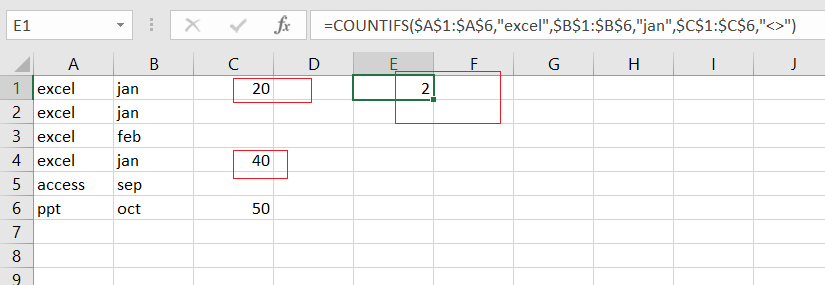# How to Count Not Empty or Blank Cells with COUNTIFS in Excel

This post will guide you how to count not empty cells or categories with COUNTIFS function in Excel 2013/2016 or Excel office 365. You can use the COUNTIFS function to count not blank cells with a given criteria in excel.

## Summary Not Empty Cells with COUNTIFS

Assuming you have a dataset in range A1:C6, and you want to create a formula which can count non-blank cells in column C. Non blank means something has not been enter. And you want column A to be equals a particular item(“excel”) and column B equals to a particular value(“2016”). You may need a specific formula, which will count the number of results for a particular data range on a particular cell. The following formula can be used to build a summary count of non-blank values:

=COUNTIFS(\$A\$1:\$A\$6,”excel”,\$B\$1:\$B\$6,”jan”,\$C\$1:\$C\$6,”<>”)As you want a particular value in range A1:A6, and a product value as “excel” is the first criteria. And you also have another date value. And you can notice that there are three conditions. Now what’s great about count is it can count with one or more condition. You just need to put the criteria range, then criteria, and next criteria.

Now for criteria range one and you should have a question in column A. and you need to ask of every cell value in this column , if those cell values are equals to “excel”.

### Related Functions

• Excel SUMPRODUCT function
The Excel SUMPRODUCT function multiplies corresponding components in the given one or more arrays or ranges, and returns the sum of those products.The syntax of the SUMPRODUCT function is as below:= SUMPRODUCT (array1,[array2],…)…
• Excel COUNTIF function
The Excel COUNTIF function will count the number of cells in a range that meet a given criteria. This function can be used to count the different kinds of cells with number, date, text values, blank, non-blanks, or containing specific characters.etc.= COUNTIF (range, criteria)…
• Excel COUNTIFS function
The Excel COUNTIFS function returns the count of cells in a range that meet one or more criteria. The syntax of the COUNTIFS function is as below:= COUNTIFS(criteria_range1, criteria1, [criteria_range2, criteria2]…)…